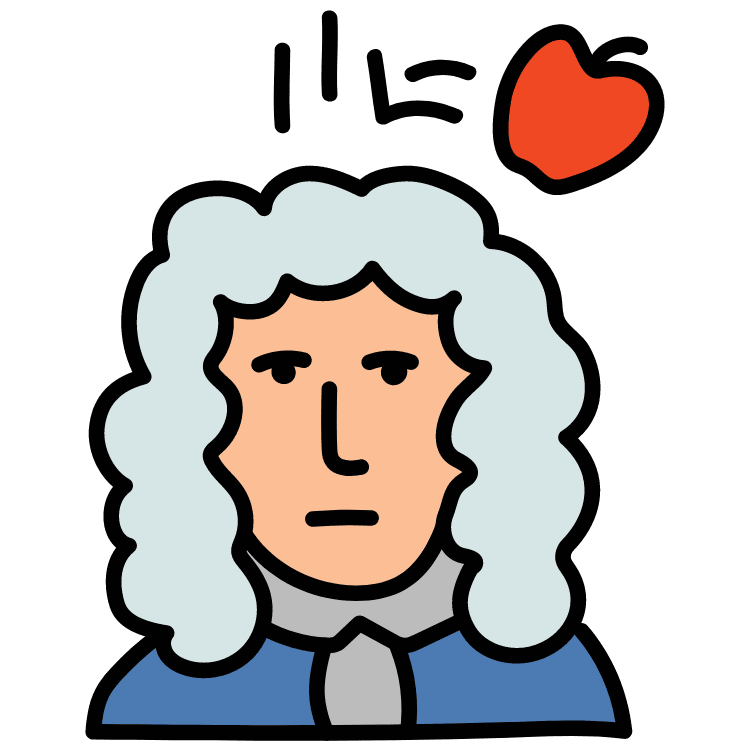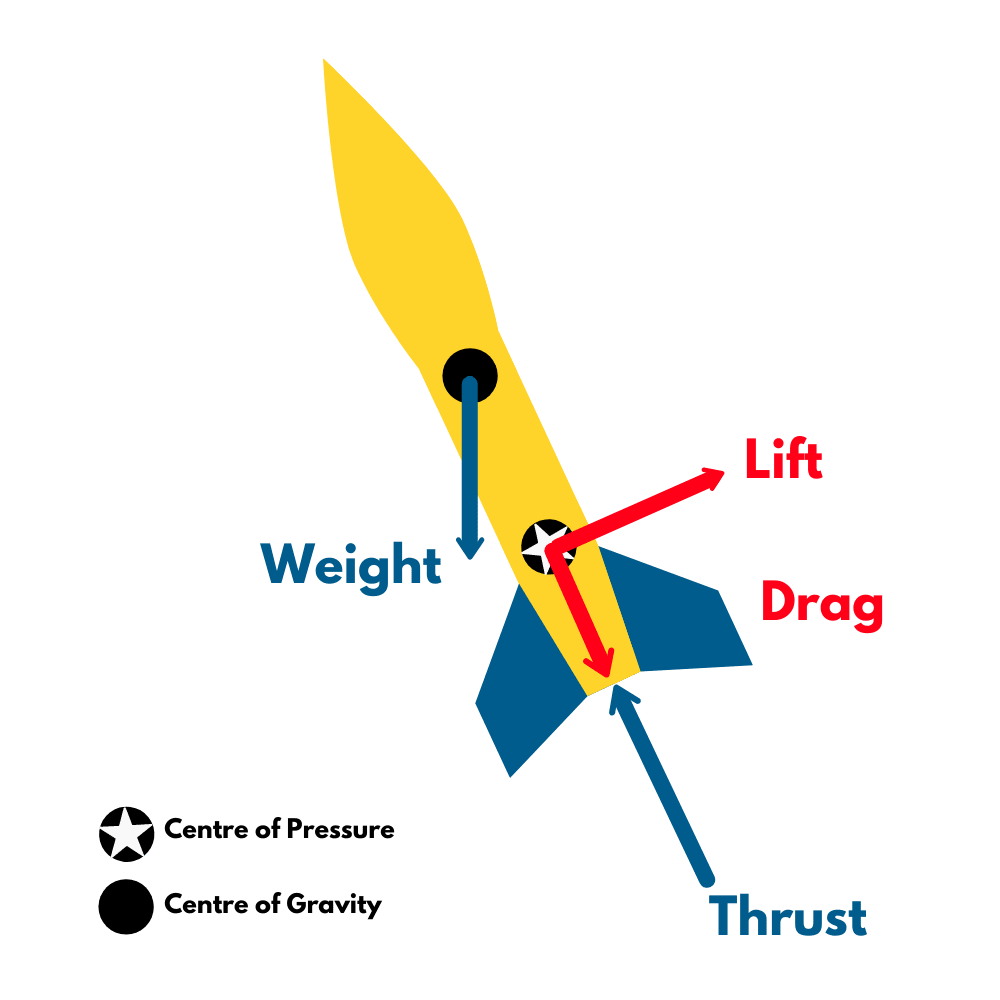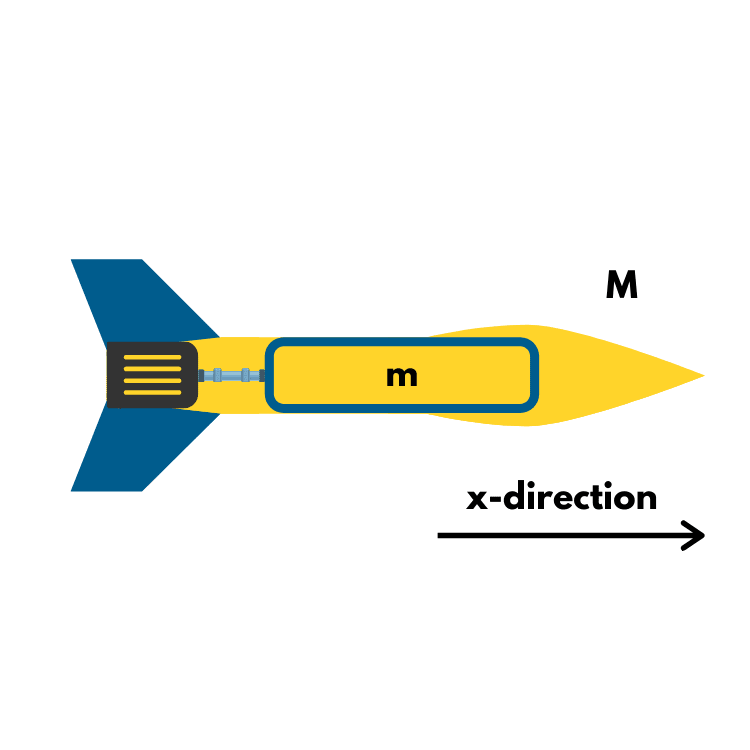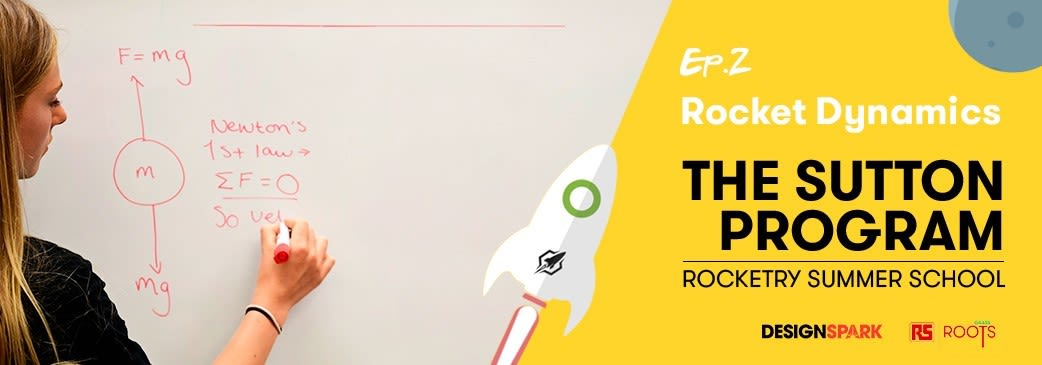# Rocket Dynamics - Sutton Program Article 2

This article will accompany the second video in our series made possible by RS Components Grass Roots, aiming to introduce key rocketry concepts and principles. This article will be looking at Sir Isaac Newton and his 3 fundamental laws of motion that govern rocket dynamics. Written by Sean Clark and Kieran Webb

### Introduction

Sir Isaac Newton is known worldwide for his work on physics. Most commonly due to his 3 fundamental laws governing the motion of an object. Despite living 300 years ago, his laws are still taught at the foundation of physics today, and it is these laws that must be explored further to better understand the forces involved in rocket flight.The three laws in question were first published in a very important piece of scientific literature in 1687: Newton’s Principia Mathematica. This publication covers a range of topics from orbits of celestial bodies, mass estimates of the sun and Earth, hydrostatics, foundations of modern-day calculus and Newton’s 3 fundamental laws of motion.

### First Law

Newton’s first law states:

“An object at rest will stay at rest, an object in motion will stay in motion unless acted on by a net external force.”

This law is fairly intuitive; we know that a ball would never start rolling unless it was placed upon a hill, or it was pushed. This same logic applies to all objects. If an object is stationary, it will not move unless acted on by some external force. This means that if there is no force acting on an object then the velocity of the object will remain constant.

This introduces 2 new terms, momentum and inertia. Momentum is a measure of the resistance of a body in motion to be brought to rest. Inertia is the tendency of an object to resist change to its state of motion.Inertia is the reason why bicycles and cars have gears. If a cyclist starts pedalling a bicycle in a higher gear, they will have to apply more force to see any acceleration as the inertial force they have to overcome is much greater than that of a lower gear.

We know that as the speed of the bicycle increases, the time and distance taken to come to a stop will be greater – this is due to momentum. Momentum is proportional to velocity for a constant mass so as you peddle harder on the bicycle, momentum increases, making it harder to stop. The equation for momentum is shown below.

p = m * v

The rocket is obviously not going to move itself, but these terms help to define and predict the response the rocket will have to the forces it is subjected to.

### Second Law

Newton’s second law goes into more detail covering how an external force effects the motion of an object. The law states that:

“The rate of change of momentum of an object over time is proportional to the force applied.”

This means that to change velocity (non-zero acceleration) some force must act on the body, and the effect of this force will vary depending on the mass of the object.

F = dp / dt

This equation provides the change in momentum due to a force acting on the body and hence the velocity of the object – as long as the force, magnitude and duration of action on the body are both known.

Using calculus, the above equation can be rewritten as something more familiar:

F = m * a

This shows that the force applied to an object is equal to the mass multiplied by the change in velocity also known as the acceleration. This means that the heavier the rocket, the more powerful the engines need to be in order to accelerate it. However, this relationship can only be applied to a situation in which the mass is constant- as a rocket expels mass to produce thrust, the net mass of the rocket cannot be constant.

When a rocket is exhausting combusted fuel, its mass is decreasing, but the same force is still being applied. Therefore, the acceleration must increase to balance the relationship. This is known as the conservation of momentum and is extremely important in rocket flight.

### Third Law

Newton’s third law states that:

“Every action has an equal and opposite reaction”

the body which produces the force acting on an object will receive the same magnitude of force as it applied but back upon itself. This means that across the interaction of multiple bodies, the total momentum of the system must be constant.

Individually, each law is important- however together, they govern the dynamics of a rocket.

### Four forces on a rocketA rocket is subject to a total of four main forces during flight, each of which acts within a given direction and magnitude due to a force being a vector. These forces directly affect the rocket’s behaviour during flight and adding them together allows for us to calculate the total force being exerted on the rocket. The four forces along with their corresponding symbol in brackets are as follows:

• Weight (W) is simply the mass of the rocket multiplied by ‘g’
• Thrust (T) is a force to counteract weight (in a rocket) and is produced by the engine
• Lift (L) is the aerodynamic force that provides stability to the rocket in atmospheric flight
• Drag (D) can be described as aerodynamic friction and has multiple forms

The terms in bold are explained in further detail in our ‘Rocket Aerodynamics’ section of the program.

### The Conservation of Momentum

This principle is a foundational physics concept and is extremely important in the successful operation of a rocket. Momentum within any situation cannot be created or destroyed but must remain constant, only transferred from one body to another through the application of the three laws described above.

A rocket is a great example of this principle – momentum is transferred in one direction to the rocket’s expelled mass, and thus momentum must also be transferred to the rocket as well.

We can think of a rocket as being comprised of two separate masses. We will denote the rocket’s ‘empty’ mass as 'M' , as well as denoting the rocket’s fuel mass as 'm'. In this case, the empty mass of the rocket will be much larger than the fuel mass (M>m).Together, our total system mass is equal to M + m. From the first equation, we can now write each mass’ momenta as pM and pm respectively.

In this example, we can assume the rocket to have zero external forces acting upon it. If the rocket is at rest, the momentum of the entire system is zero.

When the rocket engine fires and the combusted fuel is exhausted as a gas, momentum is given to the expelled mass in the negative x-direction. In turn, due to the conservation of momentum, momentum must now also be given to the rocket in the opposite direction.

pM = - pm

As the momentum transfer occurs in opposite directions, they will have opposing signs- one in the positive x-direction and one in the negative- and therefore cancel each other out.As the rocket is comprised of two ‘separate’ masses, one much larger than the other, their velocities must be different in order to maintain conservation of momentum. As the empty rocket has a larger mass, the principle requires it to have a lower velocity, so the fuel must have a smaller mass and therefore the higher velocity,

### The three laws in a rocket launch

Initially, the rocket is sitting on a launch pad and the first law states that it will remain there until acted upon by an external force.

The second law determines what force is needed to overcome the effects of gravity. As the gravitational force produced by the Earth is known as 9.81 ms-2, this means an engine is needed with the capacity to exert a force 9.81 times the mass of the rocket to initiate acceleration off the ground. In reality, this is the absolute minimum needed and will produce a very small acceleration, the real amount of force will be over 3 times this number to obtain a sufficient velocity.

The third law dictates what happens at take-off, how the rocket engines manage to exert a propulsive force and achieve flight. The hot gases inside the engine are ignited and expand. These are then expelled out the nozzle at very high speeds– multiple times the speed of sound. The exhausted gases have mass and velocity and therefore they are a force.

Newton’s third law states that momentum in a system must be conserved so for these gases to be able to produce a force there must also be another force of equal magnitude in the opposite direction – this is the propulsive force they create which acts on the rocket to achieve lift-off.

This has been a rundown of Newton’s fundamental laws and their application to rocketry. NASA has an excellent library of educational content for both basic and advanced physics principles related to rockets. Thanks for reading, and happy learning!

Parts in this series:We are a University of Glasgow based engineering project, focused on launching Scotland's first student-lead, high-powered rocket.